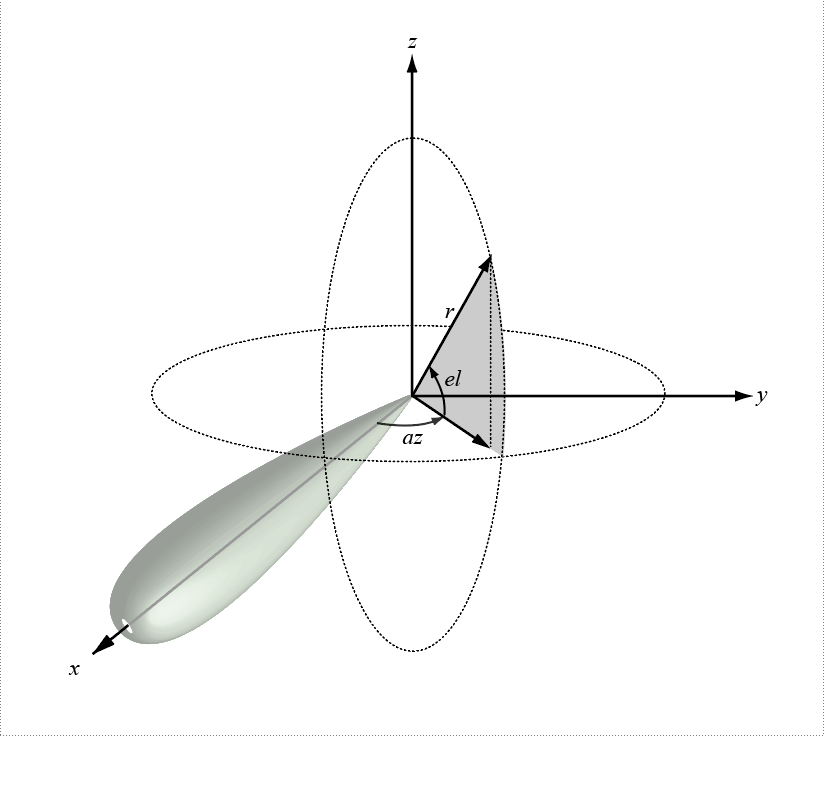Main Content

# local2globalcoord

Convert local to global coordinates

## Syntax

```gCoord = local2globalcoord(lclCoord,OPTION) gCoord = local2globalcoord(___,localOrigin) gCoord = local2globalcoord(___,localAxes) ```

## Description

`gCoord = local2globalcoord(lclCoord,OPTION)` converts local coordinates `lclCoord` to global coordinates `gCoord`. `OPTION` determines the type of local-to-global coordinate transformation.

`gCoord = local2globalcoord(___,localOrigin)` specifies the origin of the local coordinate system, `localOrigin`.

`gCoord = local2globalcoord(___,localAxes)` specifies the axes of the local coordinate system, `localAxes`.

## Input Arguments

`lclCoord`

Local coordinates in rectangular or spherical coordinate form, specified as a 3-by-N matrix. Each column represents one set of local coordinates.

If the coordinates are in rectangular form, each column contains the (x,y,z) components. Units are in meters.

If the coordinates are in spherical form, each column contains (az,el,r) components. az is the azimuth angle in degrees, el is the elevation angle in degrees, and r is the radius in meters.

`OPTION`

Types of coordinate transformations, specified as a character vector. Valid values are

OPTIONTransformation
`'rr'`Local rectangular to global rectangular
`'rs'`Local rectangular to global spherical
`'sr'`Local spherical to global rectangular
`'ss'`Local spherical to global spherical

`localOrigin`

Origin of local coordinate system, specified as a 3-by-N matrix containing the rectangular coordinates of the local coordinate system origin with respect to the global coordinate system. N must match the number of columns of `gCoord`. Each column represents a separate origin. However, you can specify `localOrigin` as a 3-by-1 vector. In this case, `localOrigin` is expanded into a 3-by-N matrix with identical columns.

Default: `[0;0;0]`

`localAxes`

Axes of local coordinate system, specified as a 3-by-3-by-N array. Each page contains a 3-by-3 matrix representing a different local coordinate system axes. The columns of the 3-by-3 matrices specify the local x, y, and z axes in rectangular form with respect to the global coordinate system. However, you can specify `localAxes` as a single 3-by-3 matrix. In this case, `localAxes` is expanded into a 3-by-3-by-N array with identical 3-by-3 matrices. The default is the identity matrix.

Default: `[1 0 0;0 1 0;0 0 1]`

## Output Arguments

 `gCoord` Glabal coordinates in rectangular or spherical coordinate form, returned as a 3-by-N matrix. The dimensions of `gCoord` match the dimensions of `lclCoord`. The origin of the global coordinate system is assumed to be located at (0, 0, 0). The global system axes are the standard unit basis vectors in three-dimensional space, (1, 0, 0), (0, 1, 0), and (0, 0, 1).

## Examples

collapse all

Convert from local rectangular coordinates to global rectangular coordinates. The local coordinate origin is a (1,1,1)

`globalcoord = local2globalcoord([0;1;0], 'rr',[1;1;1])`
```globalcoord = 3×1 1 2 1 ```

Convert local spherical coordinate to global rectangular coordinate.

`globalcoord = local2globalcoord([30;45;4],'sr')`
```globalcoord = 3×1 2.4495 1.4142 2.8284 ```

Convert two vectors in global coordinates into two vectors in global coordinates using the `global2local` function. Then convert them back to local coordinates using the `local2global` function.

Start with two vectors in global coordinates, (0,1,0) and (1,1,1). The local coordinate origins are (1,5,2) and (-4,5,7).

`gCoord = [0 1; 1 1; 0 1]`
```gCoord = 3×2 0 1 1 1 0 1 ```
`lclOrig = [1 -4; 5 5; 2 7];`

Construct two rotation matrices using the rotation functions.

```lclAxes(:,:,1) = rotz(45)*roty(-15); lclAxes(:,:,2) = roty(45)*rotx(35);```

Convert the vectors in global coordinates into local coordinates.

`lclCoord = global2localcoord(gCoord,'rr',lclOrig,lclAxes)`
```lclCoord = 3×2 -3.9327 7.7782 -2.1213 -3.6822 -1.0168 1.7151 ```

Convert the vectors in local coordinates back into global coordinates.

`gCoord1 = local2globalcoord(lclCoord,'rr',lclOrig,lclAxes)`
```gCoord1 = 3×2 -0.0000 1.0000 1.0000 1.0000 0 1.0000 ```

## More About

collapse all

### Azimuth Angle, Elevation Angle

The azimuth angle of a vector is the angle between the x-axis and the orthogonal projection of the vector onto the xy plane. The angle is positive in going from the x axis toward the y axis. Azimuth angles lie between –180 and 180 degrees. The elevation angle is the angle between the vector and its orthogonal projection onto the xy-plane. The angle is positive when going toward the positive z-axis from the xy plane. By default, the boresight direction of an element or array is aligned with the positive x-axis. The boresight direction is the direction of the main lobe of an element or array.

Note

The elevation angle is sometimes defined in the literature as the angle a vector makes with the positive z-axis. The MATLAB® and Communications Toolbox™ products do not use this definition.

This figure illustrates the azimuth and elevation angles of a direction vector.## References

 Foley, J. D., A. van Dam, S. K. Feiner, and J. F. Hughes. Computer Graphics: Principles and Practice in C, 2nd Ed. Reading, MA: Addison-Wesley, 1995.

## See Also

Introduced in R2020a

## Support

#### Bridging Wireless Communications Design and Testing with MATLAB

Download white paper